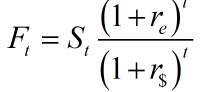# Suppose that the spot price of the euro is currently \$1.10. The 1-year futures price is \$1.15. Is the interest rate higher in the United States or the euro zone?

Question
2 views

Suppose that the spot price of the euro is currently \$1.10. The 1-year futures price is \$1.15. Is the interest rate higher in the United States or the euro zone?

check_circle

Step 1

The question is based on the relation between exchange rate and interest rate differential between countries. The relationship explains in form of Interest rate parity (IRP) and forward exchange rate .

Step 2

The spot price of the euro is currently \$1.10 and the 1-year futures price is \$1.15. The relation of future exchange rate, spot rate and interest rate can be explained byCase 1: if interest rate in Euro (re )= interest rate in Dollar (r\$ ) , then Future rate(Ft) = Spot rate(St) at time (t) of 1 year

Case 2: if interest rate in Euro (re )> interest rate in Dollar (r\$ ) , then Future rate(Ft) > Spot rate(St) time (t) of 1 year

Case 3:  if interest rate in Euro (re)< interest rate in Dollar (r\$) , then Future rate(Ft) < Spot rate(St) time...

### Want to see the full answer?

See Solution

#### Want to see this answer and more?

Solutions are written by subject experts who are available 24/7. Questions are typically answered within 1 hour.*

See Solution
*Response times may vary by subject and question.
Tagged in